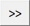# TerraScan User Guide

 Navigation: Batch Processing Reference > Classification Routines > Points By normal vector

By normal vector

Not Lite

By normal vector routine classifies points based on normal vector values and/or dimension types.

Normal vector and dimension can be stored as attributes for points in TerraScan FastBinary files. They must be computed by using the Compute normal vectors command before starting the classification routine.

The normal vector is computed for points with planar dimension. It contains three components, X, Y, and Z. Points can be classified by using the dimension attribute only, or by specifying certain normal vector components and their values. The values can range from -1.0 to +1.0 for each component. For instance, the value +1.0 for the Z component means that the normal vector points upward from a completely horizontal surface.

The routine is useful, for example, to classify points on very flat parts of a road surface in a mobile data set or to classify points on roof planes facing to a certain direction in an airborne data set.SETTING

EFFECT

From class

Source class(es).Opens the Select classes dialog which contains the list of active classes in TerraScan. You can select multiple source classes from the list that are then used in the From class field.

To class

Target class.

Dimension

Dimension of points to be classified: Not known, Linear, Planar, or Complex. See Compute normal vectors command for a more detailed description of dimensions.

X

If on, points are classified, if the X component of their normal vector falls within the given range of values.

Y

If on, points are classified, if the Y component of their normal vector falls within the given range of values.

Z

If on, points are classified, if the Z component of their normal vector falls within the given range of values.

Inside fence only

If on, points inside a fence or selected polygon(s) are classified.

TerraScan User Guide   26.07.2021   © 2021 Terrasolid Ltd